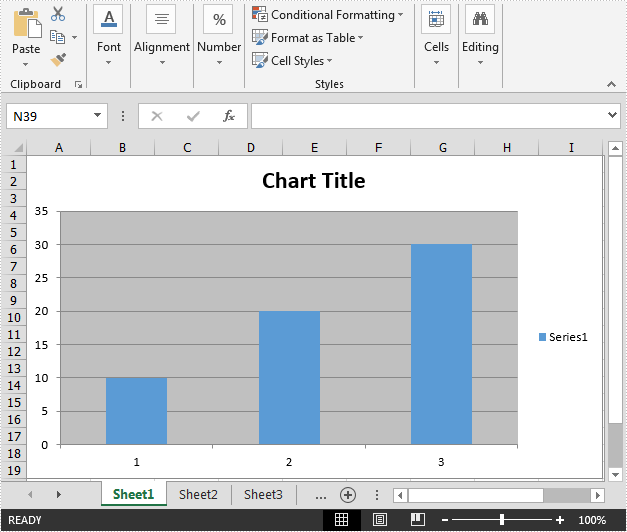Friday, 15 February 2019 08:27

## Create Chart without Using Worksheet Data Range in C#

Written by  support iceblue
Rate this item

This article demonstrates how to create a chart without reference to the worksheet data range using Spire.XLS.

Detail steps:

Step 1: Create a workbook and get the first worksheet.

```Workbook wb = new Workbook();
Worksheet sheet = wb.Worksheets;
```

Step 2: Add a chart to the worksheet.

```Chart chart = sheet.Charts.Add();
```

Step 3: Add a series to the chart.

```var series = chart.Series.Add();
```

```series.EnteredDirectlyValues = new object[] { 10, 20, 30 };
```

Step 5: Save the file.

```wb.SaveToFile("result.xlsx", ExcelVersion.Version2013);
```

Output:Full code:

```using Spire.Xls;

namespace Create_chart
{
class Program
{
static void Main(string[] args)
{
//Create a workbook
Workbook wb = new Workbook();

//Get the first worksheet
Worksheet sheet = wb.Worksheets;

//Add a chart to the worksheet

//Add a series to the chart

series.EnteredDirectlyValues = new object[] { 10, 20, 30 };

//Save the file
wb.SaveToFile("result.xlsx", ExcelVersion.Version2013);
}
}
}
```